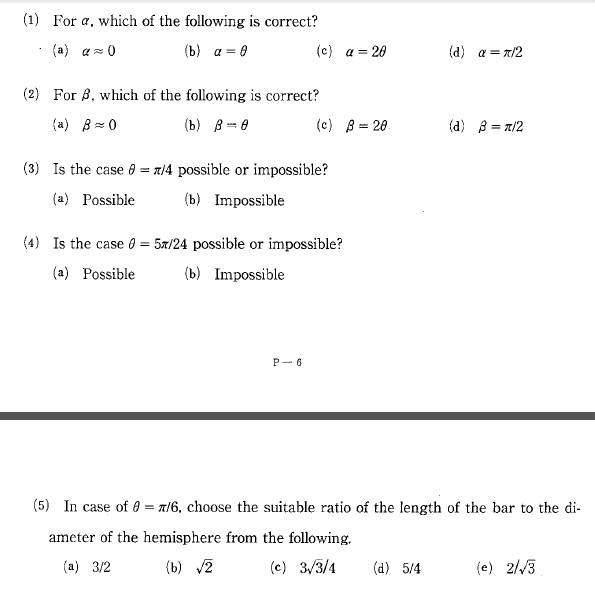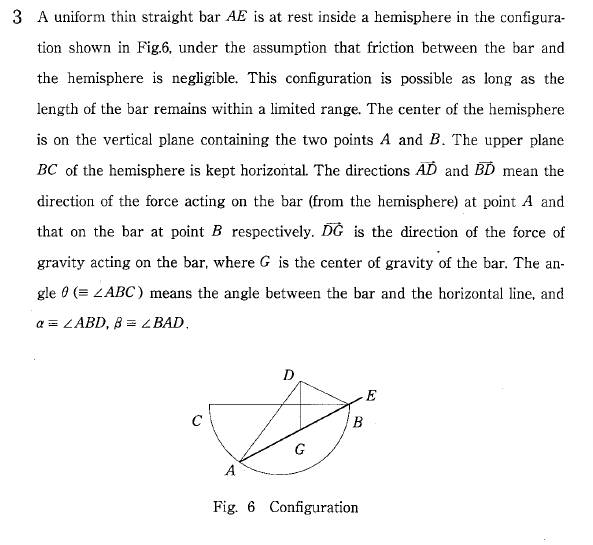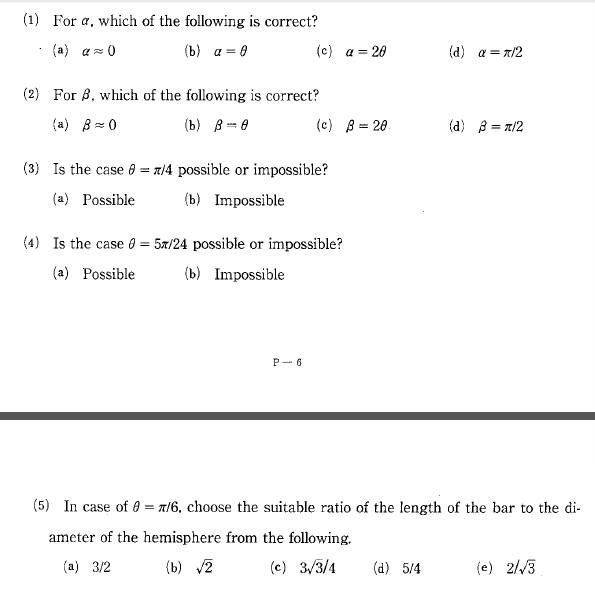# Equilibrium of forces

• thevinciz

## Homework Statement

The questions are in the comment box. Sorry!
https://www.img.in.th/image/V9K8Rg## The Attempt at a Solution

1. I think α = 90 because BD is normal force.

and I don't know anymore

#### Attachments

Last edited by a moderator:#### Attachments

Looks like we tried to fix the images at the same time.

1. I think α = 90 because BD is normal force.
Normal to what? What does that tell you about the geometry?

Looks like we tried to fix the images at the same time.

Normal to what? What does that tell you about the geometry?

I think it is a normal force (from hemisphere) acting on the bar, so ABD which is angle of normal force should be 90 degree.

I agree.

Can you answer (2) now? Hint: Draw a good diagram, to scale if you can.

Can you answer (2) now? Hint: Draw a good diagram, to scale if you can.

If I call a point (located at middle of BC) O, I think OA and OB are radius. Because OA and OB have same length so BAD = ABC. Is this correct?

Last edited:
If I call a point (located at middle of BC) O, I think OA and OB are radius. Because OA and OB have same length so BAD = ABC. Is this correct?
Right, but exactly what is your reasoning?

Right, but exactly what is your reasoning?

I'm sorry I don't understand what you mean

I'm sorry I don't understand what you mean
You are right that BAD=ABC, but I do not see how you deduced that. Might be something simple I am missing.

OA and OB having the same length tells you that BAO and ABO=ABC are the same. There is a step missing.

You are right that BAD=ABC, but I do not see how you deduced that. Might be something simple I am missing.

AD and BD are normal force which are perpendicular to a tangent line of hemisphere. Therefore AO and BO are radius of the hemisphere.

OA and OB having the same length tells you that BAO and ABO=ABC are the same. There is a step missing.

I just explain it above this comment, could you check it please?

Could you guys help me the question 3-5 please

AD and BD are normal force which are perpendicular to a tangent line of hemisphere. Therefore AO and BO are radius of the hemisphere.
Ah yes, I see. Except, BD is not perpendicular to the tangent. It is perpendicular to the rod.

Could you guys help me the question 3-5 please
For 3, try to draw the diagram specific to that case.
Where is A now?

Ah yes, I see. Except, BD is not perpendicular to the tangent. It is perpendicular to the rod.

Thanks , I made a mistake

Could you guys help me the question 3-5 please
The key to this problem is this. Consider the point of intersection of segments AD and CE. What's special about it? Then either draw to-scale diagrams specific to each case as @haruspex suggested or derive a general expression for the ratio of the length to diameter in terms of angle ##\theta## and see what it is for each angle.

Consider the point of intersection of segments AD and CE.
I believe thevinciz did that in determining that OA and OB are radii.

I believe thevinciz did that in determining that OA and OB are radii.

Yes OD is interesting, but please see my edit to post #19.

I believe thevinciz did that in determining that OA and OB are radii.
I read the edit. I meant the intersection of AD and CB. Note that the normal force at A is centripetal ...

For the question3, I have found that if θ=45° (β=45° too because β=θ) this configuration is impossible.

Because we will have AOB = 90° and the nearby angle 90° (the angle of above triangle that is located on AD) but the above triangle has 90° angle already, so the other angle will be 0°. Therefore it is impossible.

Is this correct. Sorry for bad English again. I'm trying my best

I meant the intersection of AD and CB
I was hoping you meant that. Thanks for confirming.

we will have AOB = 90°
Yes.
the angle of above triangle that is located on AD
You've lost me. Can you explain using the names of the points? Name some more points if necessary.

Yes.

You've lost me. Can you explain using the names of the points? Name some more points if necessary.

I'm trying to insert a picture, sorry.

I'm trying to insert a picture, sorry.
Can you just say where it puts D in relation to the rod?

Can you just say where it puts D in relation to the rod?

I don't know

I don't know
We have already established that BD is perpendicular to the rod. This means that triangle ABD is a right triangle with hypotenuse AD. How is AD related to the diameter of the circle? You may not be able to see what's going on unless you draw a good diagram as I have repeatedly suggested. The one provided to you appears to be deliberately misshapen perhaps so that nothing is given away. Try redrawing it, making the circle as round as possible and the perpendiculars as "perpendicular" as possible.

We have already established that BD is perpendicular to the rod. This means that triangle ABD is a right triangle with hypotenuse AD. How is AD related to the diameter of the circle? You may not be able to see what's going on unless you draw a good diagram as I have repeatedly suggested. The one provided to you appears to be deliberately misshapen perhaps so that nothing is given away. Try redrawing it, making the circle as round as possible and the perpendiculars as "perpendicular" as possible.

Oh I see, thanks you very much, I'll try draw it.

We have already established that BD is perpendicular to the rod. This means that triangle ABD is a right triangle with hypotenuse AD. How is AD related to the diameter of the circle? You may not be able to see what's going on unless you draw a good diagram as I have repeatedly suggested. The one provided to you appears to be deliberately misshapen perhaps so that nothing is given away. Try redrawing it, making the circle as round as possible and the perpendiculars as "perpendicular" as possible.

I have drawn it, AD is a diameter?

Yes! Based on that knowledge, what kind of triangle is BOD? What are its 3 angles in relation to ##\theta##?

Yes! Based on that knowledge, what kind of triangle is BOD? What are its 3 angles in relation to ##\theta##?

But I still don't know how to find AE to answer question5, could you give me more hint?

Those 3 angles are 60°
No, it is not necessarily equilateral. BD need not equal the radius.
It would help to find the answer to my question in post #27.
If exactly three forces act on a body in equilibrium, what can you say about the lines of action of the three forces? Consider moments.